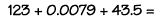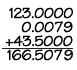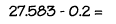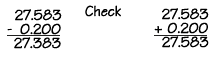Home    |    Teacher    |    Parents    |    Glossary    |    About UsIf you know how to add and subtract whole numbers, then you can add and subtract decimals! Just be sure to line up the terms so that all the decimal points are in a vertical line.

1. Put the numbers in a vertical column, aligning the decimal points
2. Add each column of digits, starting on the right and working left. If the sum of a column is more than ten, "carry" digits to the next column on the left.
3. Place the decimal point in the answer directly below the decimal points in the terms.

To subtract decimal numbers:

1. Put the numbers in a vertical column, aligning the decimal points.
2. Subtract each column, starting on the right and working left. If the digit being subtracted in a column is larger than the digit above it, "borrow" a digit from the next column to the left.
3. Place the decimal point in the answer directly below the decimal points in the terms.
4. Check your answer by adding the result to the number subtracted. The sum should equal the first number.

Let's look at an example.To add these numbers, first arrange the terms vertically, aligning the decimal points in each term. Don't forget, for a whole number like the first term, the decimal point lies just to the right of the ones column. You can add zeroes to the right of the decimal point to make it easier to align the columns. Then add the columns working from the right to the left, positioning the decimal point in the answer directly under the decimal points in the terms.Here's a subtraction example.To subtract these numbers, first arrange the terms vertically, aligning the decimal points in each term. You can add zeroes to the right of the decimal point, to make it easier to align the columns. Then subtract the columns working from the right to the left, putting the decimal point in the answer directly underneath the decimal points in the terms. Check your answer by adding it to the second term and making sure it equals the first.Homework Help | Pre-Algebra | NumbersEmail this page to a friendSearch·  Place value·  Decimal numbers·  Estimating and     rounding·  Adding / subtracting     decimals·  Multiplying decimals·  Dividing decimals·  Percent·  Exponents·  Square roots·  Signed integers·  Adding and     subtracting integers·  Multiplying and     dividing integers·  Properties of integersFirst Glance In Depth Examples WorkoutAdding and subtracting decimals